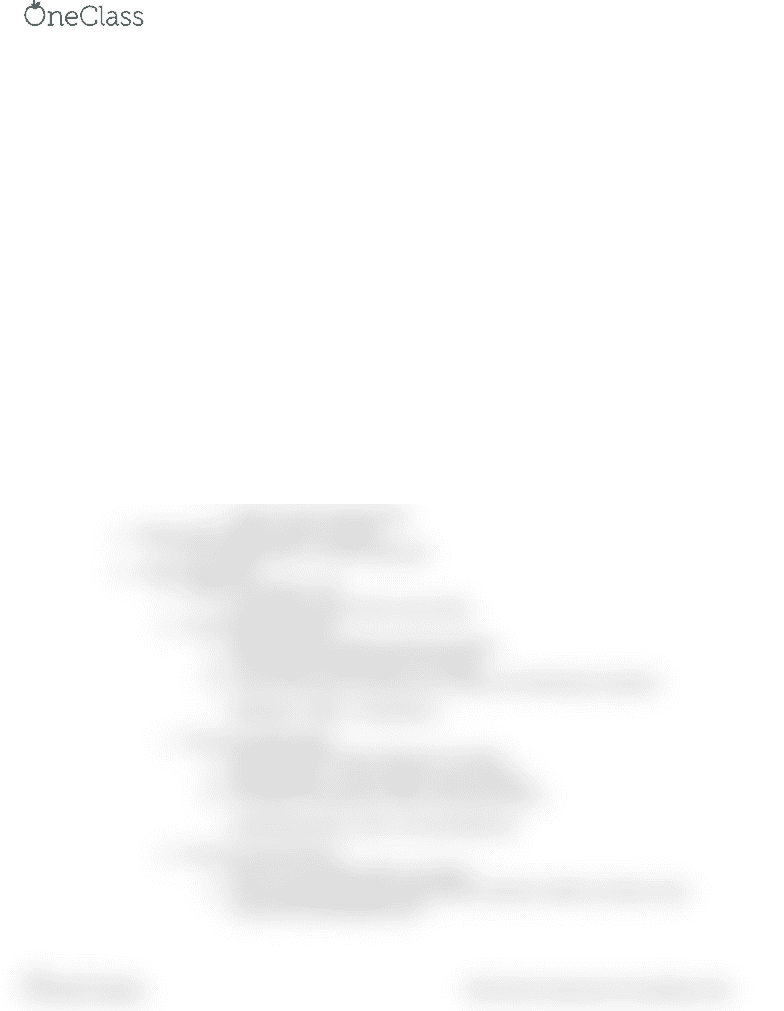# CHEM 1210 Lecture Notes - Lecture 5: Alcohol Proof, Sodium Chloride, Glucose

18 views2 pages
School
TNTECH
Department
Chemistry
Course
CHEM 1210
ProfessorLecture Outline 5: Solutions
1. Solutions- a solution is a homogenous mixture of two or more substances
a. Solute- usually less; it’s dissolved by the solvent
i. Ex) Sugar; Medicine
b. Solvent- usually more; dissolves solute
i. Ex) water
c. Mass of the solute + mass of the solvent = mass of the solution
d. Concentration of a solution refers to the amount of solute in a given amount of solution
i. Dilute: less solute
ii. Concentrate: more solute
2. Types of Solutions
a. Gas in liquid
i. oxygen in blood
b. Solid in liquid
i. sugar in water
c. Liquid in liquid
i. Alcohol in water
d. Gas in solid
i. Hydrogen in platinum
e. Liquid in solid
i. Mercury in silver
f. Solid in solid
i. Copper in silver (sterling silver)
3. Molarity (M)- moles of solute/L of solution
a. Ex.) 5.0 M NaCl (aq) = 5.0 mol/ L NaCl (aq)
4. Percent Composition
a. Percent = Part/whole x 100
i. Rearranges as percent/ 100 = part/ whole
b. Percent by weight/volume
i. Most frequently used form of percent solution
ii. % (w/v) grams of solute/ 100 mL of solution
iii. Ex) how many grams of dextrose are in 100 mL of a 50% dextrose solution?
50g/100mL x 100mL = 50 g dextrose
c. Percent by weight/weight
i. %(w/w)= grams of solute/ 100 grams of solution
ii. Mass of solution = mass of solute + mass of solvent
iii. Ex) 3g NaCl and 15g water. What is the percent (w/w)?
3g NaCl/18g solution x 100 = 17% (w/w) NaCl (aq)
d. Percent by volume/volume
i. %(v/v)= mL of a solute/ 100 mL of solution
ii. Ex) if an alcoholic beverage contains 50mL of alcohol in 100mL of solution, what
is the %(v/v) and alcoholic proof?
find more resources at oneclass.com
find more resources at oneclass.com
Unlock document

This preview shows half of the first page of the document.
Unlock all 2 pages and 3 million more documents.